# Understanding How-to Make a Step Chart in Excel Using Index and Match Functions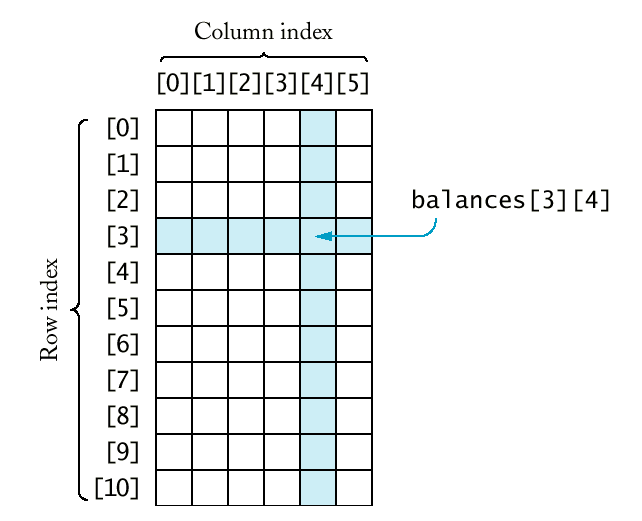Here is a quick demonstration on the technique that Peter used for his Step Charts:

To try it yourself as you watch the video, download this file and copy the formulas below:

Step Chart Data File

Video Demonstration for this technique:

In Cell C3, Peter used an Array formula to create his step chart date data

=INDEX(Table13[Date],MATCH(INT(ROW(1:1)/2)+1,ROW(Table13[Date])-ROW(\$A\$3)+1,0))

Enter this formula in cell C3 and then press CTRL+SHIFT+ENTER instead of just enter.  It will then look like this in the formula bar:

{=INDEX(Table13[Date],MATCH(INT(ROW(1:1)/2)+1,ROW(Table13[Date])-ROW(\$A\$3)+1,0))}

If you want to see how this formula is working, go to your Formula Ribbon and press the Evaluate Formula button: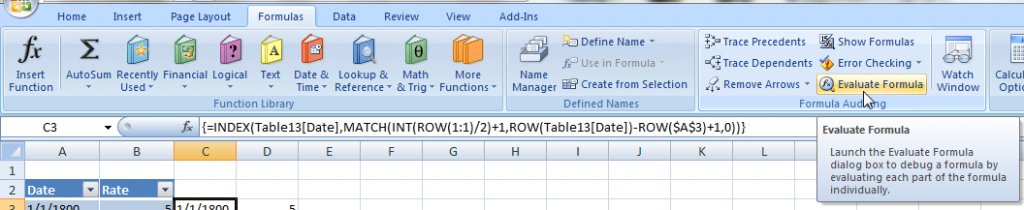And jump thru the formula by pressing the Evaluate button and see that it is doing.  You will learn a ton!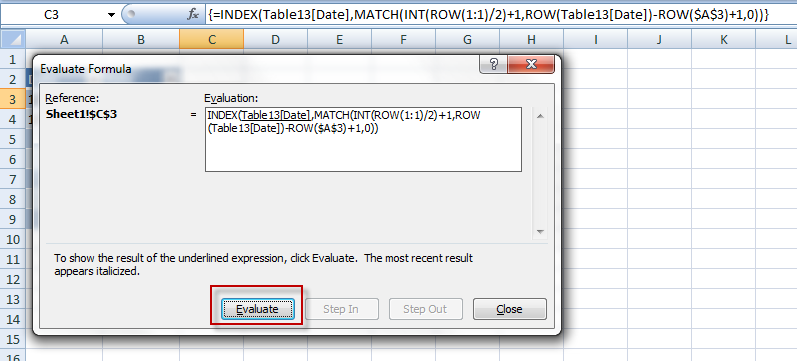In Cell D3, for the rate data, Peter used a combination of index and match and the INT function to act as a counter that steps twice (i.e. 1,2,2,3,3,4,4,5,5,6,6,etc)

Enter this formula in cell D3, but no need to press CTRL+SHIFT+ENTER, just hit enter:

=IF(INT(ROW(1:1)/2)+1<>INT(ROW(2:2)/2)+1,INDEX(Table13[Rate],MATCH(C3,Table13[Date],0)),D2)

# If you found the website and tutorials helpful, please consider donating to keep the lights on.

## Donate with PayPal here:Then copy down the data to about row 700 where you will see #NA in several rows.  Delete those rows.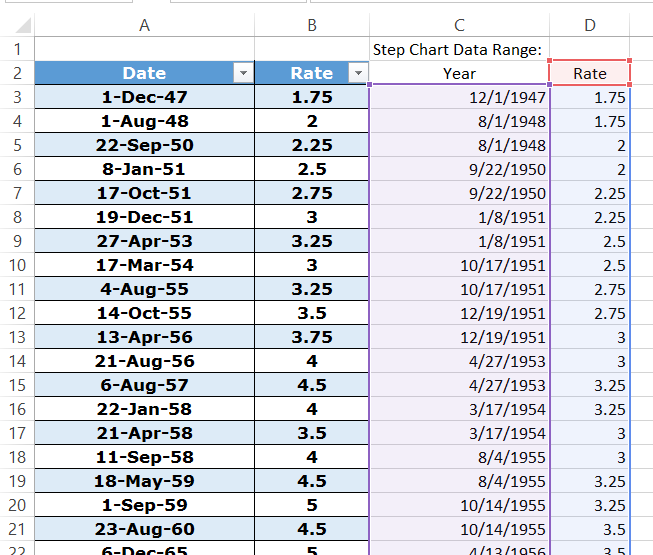Then Highlight cells C2:D683 and Insert a line chart.You will now have your fancy step chart all done for you.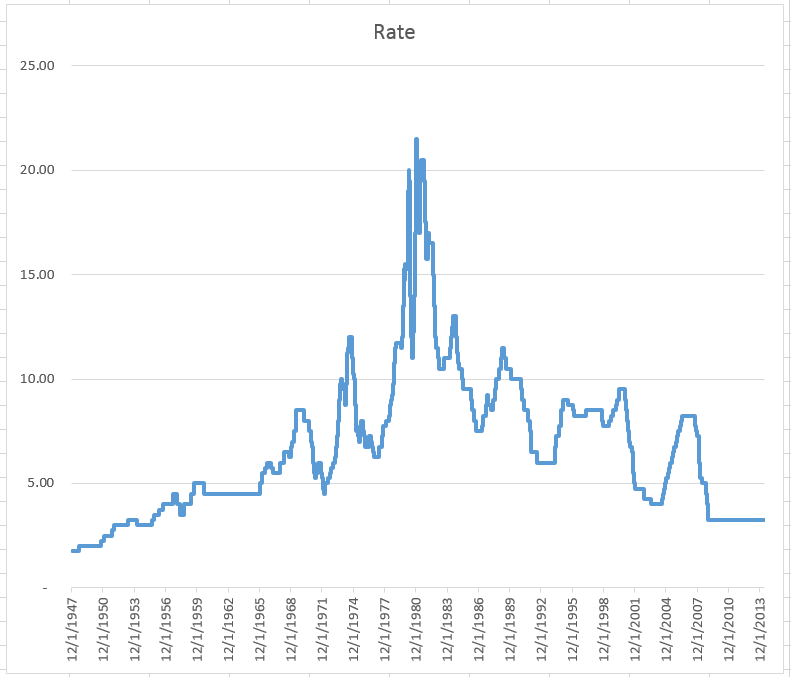Just remember, there is never one right way in Excel.  Thanks Pete for showing us another way.
Steve=True